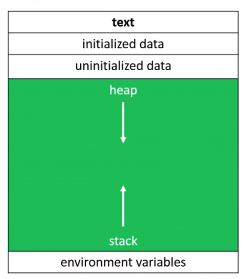# CS 50 Dynamic Memory Allocationthe variable we need to named, mostly are at the stack.
Dynamic allocated memory comes from a pool of memory known as the heap.
Prior to this point, all memory we've been working with has been coming from a pool of memory known as the stack.

// statically obtain an integer
int x;
// dyanmically obtain an integer
int *px = malloc(4); // 8 * 4 = 32;   4bytes 32int
// malloc(sizeof(int));

// array of floats on the stack
float stack_array[x];

// array of floats on the heap
float* heap_array = malloc(x * sizeof(float));


if you use dynamic memory allocation, you may use free(), or it will result memory leak.

char* word = malloc(50 * sizeof(char));
// do stuff with word

// now we're done working with that block
free(word);

#cs50 #heap #stack #pointer